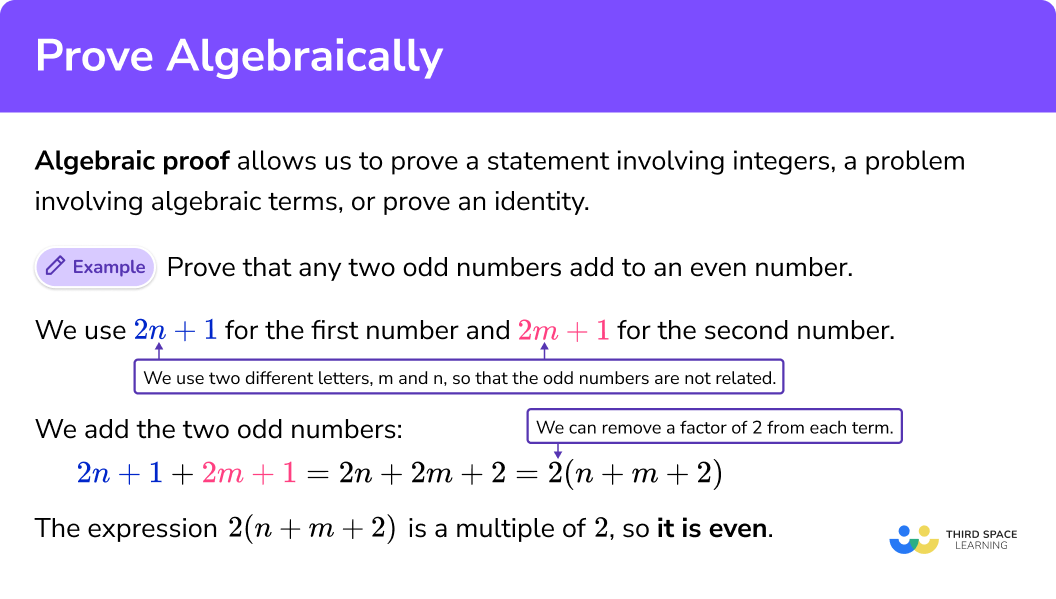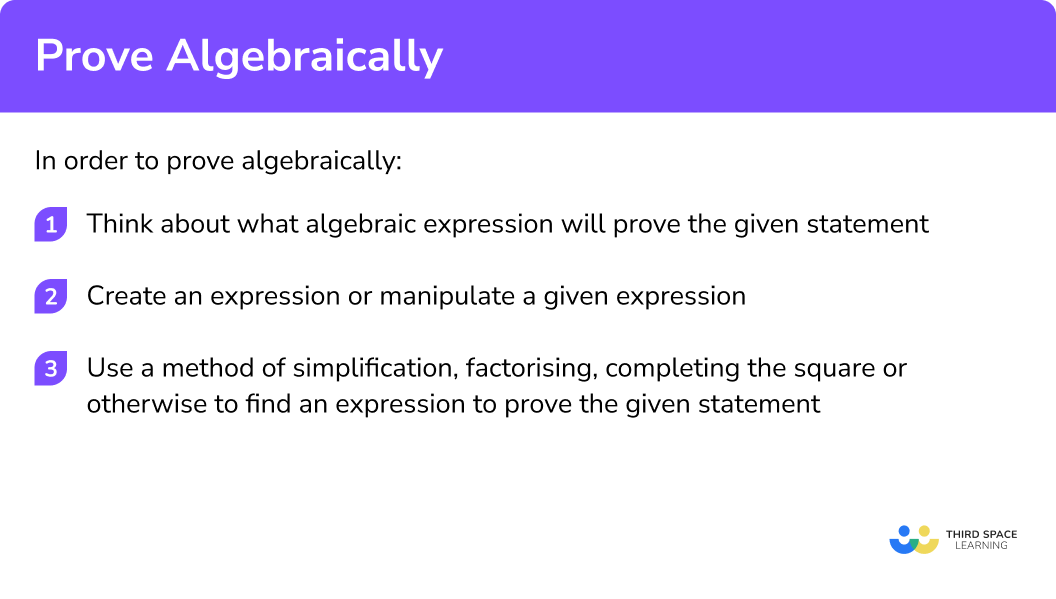GCSE Maths Algebra Proof Maths

Prove Algebraically

# Prove Algebraically

Here we will learn about proving algebraically, including proving expressions involving integers that are even, odd or multiples of other numbers, and proving expressions with positive or square numbers.

There are also prove algebraically worksheets based on Edexcel, AQA and OCR exam questions, along with further guidance on where to go next if you’re still stuck.

## What does prove algebraically mean?

Prove algebraically is a phrase used when you need to prove a statement involving integers, a problem involving algebraic terms, or prove an identity.

You will be used to seeing equations in maths, written with an equals sign (=), such as in the equation x+2=5. An equation is only true for certain values of the variable – here, the statement x+2=5 is only true for x=3.

An identity is true for any value of the variable. For example, 2x+4x is always equal to 6x, no matter what values of x are chosen, so you could write this using the identity symbol (\equiv) as 2x+4x \equiv 6x.

In order to prove that the sum of any two odd numbers is an even number, you cannot just pick a few numerical examples and show it works,

1+3=4, \ 5+7=12.

This is because you need to show that it works for every pair of odd numbers, instead of just the examples you’ve chosen.

To do this you need to know how to express different types of integers algebraically.

You can let any integer be represented by a letter. You can use any letter you want.

For algebraic proof we tend to use the letter n and may also use m if we need a second letter, but you can also use x, y, w, etc.

Even numbers

If you want to express an even number you can call it 2n, because all even numbers are multiples of 2. A multiple of 3 would be 3n, a multiple of 4 would be 4n etc..

Odd numbers

The odd numbers are always 1 above or below an even number. So you could call an odd number 2n+1.

Consecutive integers

Consecutive means “one after the other”.

If you let n be any integer, the next integer will be n+1. The one before n will be n-1

… , n-2, \ n-1, \ n, \ n+1, \ n+2, ... are consecutive integers.

… , 2n-4, \ 2n-2, \ 2n, \ 2n+2, \ 2n+4, ... are consecutive even numbers.

… , 2n-5, \ 2n-3, \ 2n-1, \ 2n+1, \ 2n+3, ... are consecutive odd numbers.

Let’s look at an example.

Prove that any two odd numbers add to an even number.

You need to have two different algebraic odd numbers. For this you should use two different letters so that the odd numbers are not related.

Let’s use 2n+1 and 2m+1.

To show that this is even, you just need to show that this expression is a multiple of 2.

To do that, show you can remove a factor of 2 from each term.

2n+2m+2\equiv 2\left( n+m+1 \right)

So because you can factor out 2, it means the expression must be even.

Therefore, you have proved algebraically that the sum of two odd numbers is always even.

Step-by-step guide: Mathematical proof

### What does prove algebraically mean?## How to prove algebraically

In order to prove algebraically:

1. Think about what algebraic expression will prove the given statement.
2. Create an expression or manipulate a given expression.
3. Use a method of simplification, factorising, completing the square or otherwise to find an expression to prove the given statement.

### Explain how to prove algebraically## Prove algebraically examples

### Example 1: prove an identity

Prove that {{\left( 5n+2 \right)}^{2}}-{{\left( 4n+5 \right)}^{2}}\equiv {{\left( 3n-4 \right)}^{2}}+4n-37

1. Think about what algebraic expression will prove the given statement.

You need to expand the brackets on the left and collect terms. You then need to separate the terms to give the expression equivalent to {{\left( 3n-4 \right)}^{2}}+4n-37

{{\left( 3n-4 \right)}^{2}}\equiv 9{{n}^{2}}-24n+16

2Create an expression or manipulate a given expression.

Expand the left hand side.

\begin{aligned} {{\left( 5n+2 \right)}^{2}}-{{\left( 4n+5 \right)}^{2}}& \equiv \left( 25{{n}^{2}}+20n+4 \right)-\left( 16{{n}^{2}}+40n+25 \right) \\\\ & \equiv 9{{n}^{2}}-20n-21 \end{aligned}

3Use a method of simplification, factorising, completing the square or otherwise to find an expression to prove the given statement.

Use the expanded expression for {{\left( 3n-4 \right)}^{2}}\equiv 9{{n}^{2}}-24n+16 to find the remaining required terms.

\begin{aligned} 9{{n}^{2}}-20n-21 & \equiv 9{{n}^{2}}-24n+16+4n-37 \\\\ & \equiv {{\left( 3n-4 \right)}^{2}}+4n-37 \end{aligned}

### Example 2: prove that an algebraic expression is a multiple of an integer

Prove that {{\left( 2n+3 \right)}^{2}}-{{\left( n-3 \right)}^{2}} is a multiple of 3, where n is an integer.

Think about what algebraic expression will prove the given statement.

Create an expression or manipulate a given expression

Use a method of simplification, factorising, completing the square or otherwise to find an expression to prove the given statement.

### Example 3: prove a worded statement algebraically

Prove that the difference of two consecutive square numbers is odd.

Think about what algebraic expression will prove the given statement.

Create an expression or manipulate a given expression

Use a method of simplification, factorising, completing the square or otherwise to find an expression to prove the given statement.

### Example 4: prove a worded statement algebraically

Prove that the sum of four consecutive odd numbers is a multiple of 8.

Think about what algebraic expression will prove the given statement.

Create an expression or manipulate a given expression

Use a method of simplification, factorising, completing the square or otherwise to find an expression to prove the given statement.

### Common misconceptions

• Incorrect expression for odd and even integers

A common mistake is to write n and n+1 for even and odd integers. It is important to remember that even numbers are multiples of 2 \ (2n) and odd numbers are an even number plus any odd number (2n-1).

• Errors when expanding squared brackets

For example, when expanding {\left( 2n-1 \right)}^{2} remember that this means (2n-1)(2n-1), and not just to square 2n and square -1.

• Errors with signs when expanding squared brackets

For example, when expanding (n-1)^{2}, remember that {\left( -1 \right)}^{2}=1 and not -1.

### Practice prove algebraically questions

1. Which is an expression for an odd number for any value of n?

n+12n+1n-12nAn odd number is one more or less than a multiple of 2.

2. What could be the correct expressions for two consecutive even numbers for any value of n?

2n, \ 2n+2n, \ n+22n-1, \ 2n+1n-2, \ nAn even number can be written as 2n. The next even number will be 2 more.

3. What could be the correct expression for the sum of two consecutive square numbers?

2{{n}^{2}}+12{{n}^{2}}+2n2{{n}^{2}}+2n+1{{n}^{2}}+n+1{{n}^{2}}+{{\left( n+1 \right)}^{2}}\equiv {{n}^{2}}+{{n}^{2}}+2n+1

4. The sum of the squares of two consecutive numbers is definitely…

EvenA multiple of 4A multiple of 3Odd\begin{aligned} {{n}^{2}}+{{\left( n+1 \right)}^{2}} & \equiv 2{{n}^{2}}+2n+1 \\\\ & \equiv 2\left( {{n}^{2}}+n \right)+1 \end{aligned}

Therefore, the sum of two consecutive square numbers is odd.

5. A quadratic expression can be written as {{\left( n-3 \right)}^{2}}+1. What can be said about the original expression?

Always negativeAlways positiveAlways oddAlways even{{\left( n-3 \right)}^{2}}\ge 0, \ {{\left( n-3 \right)}^{2}}+1\ge 1, therefore the original expression will always be positive. This is because the square of any (real) number is positive.

6. The sum of two consecutive numbers is always…

EvenPrimeOddA multiple of 3n+n+1=2n+1

### Prove algebraically GCSE questions

1. Prove that the difference between the squares of two consecutive integers is equal to the sum of the two integers.

(3 marks)

Expressions for two consecutive integers in the form n, \ n+1.

(1)

The expression for the difference of the square given {{\left( n+1 \right)}^{2}}-{{n}^{2}}.

(1)

Evidence that the difference is equal to 2n+1.

(1)

2. (a) Prove the identity

{{\left( 3n+1 \right)}^{2}}-{{\left( 3n-1 \right)}^{2}}\equiv 12n.

(b) Hence, prove that {{\left( 3n+1 \right)}^{2}}-{{\left( 3n-1 \right)}^{2}} is always a multiple of 4 for positive integer values of n.

(4 marks)

(a)

9{{n}^{2}}+6n+1 or 9{{n}^{2}}-6n+1

(1)

Attempted subtraction shown.

(1)

Evidence of 12n found.

(1)

(b)

12n=4(3n), and statement given.

(1)

3. Prove that the difference between any two even square numbers will be a multiple of 4.

(3 marks)

2n and 2m .

(1)

4{{n}^{2}}-4{{m}^{2}}

(1)

4\left( {{n}^{2}}-{{m}^{2}} \right)

(1)

## Learning checklist

You have now learned how to:

• Understand the difference between an equation and an identity
• Argue mathematically to show algebraic expressions are equivalent
• Use algebra to support and construct arguments and proofs

## Still stuck?

Prepare your KS4 students for maths GCSEs success with Third Space Learning. Weekly online one to one GCSE maths revision lessons delivered by expert maths tutors.

Find out more about our GCSE maths tuition programme.